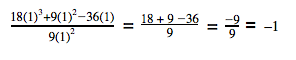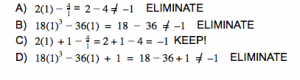# SAT Math: Top 6 Tips for the No-Calculator Section

Over the years, you may have become accustomed to using a calculator to solve each and every math question. A straightforward calculation you could do in third grade, such as 7 x 12, may have you reaching for your calculator. After all, pushing just a few buttons on a calculator will get you to the answer (it’s 84, by the way). It’s time to awaken that part of your brain from its possibly lengthy slumber because the new SAT will require you to work through the first Math section without a calculator. But don’t worry! Follow these six tips to help you ace the SAT No-Calculator Math Test!

### SAT Math Tip #1: Know the test so you can pace yourself

The No-Calculator Math Test is the third multiple-choice section of the SAT. You will have 25 minutes to answer 15 multiple choice questions and 5 grid-in questions, leaving you with a little more than a minute per question.
For a closer look at content tested, see What’s Tested on the SAT Math Section.

### SAT Math Tip #2: Think first, then compute

The No-Calculator Math questions will not require long, drawn-out calculations. Remember: these questions are all designed to take less than a minute to solve. Look for patterns and shortcuts to solve complicated-looking questions more easily. For instance, consider the question below:This should bring back memories from Algebra I and II when you had to solve a system of equations. Before you start solving for one of the variables in the first equation to substitute into the second equation, take a moment to notice the structure of the equations. The equations are formatted perfectly for combination. Combination (sometimes referred to as elimination) is used to solve systems of equations; you eliminate one variable by adding the equations, and then you solve for the remaining variable.
In this case, when you add 4x + y = –5 to –4x – 2y = –2, the x values add up to 0, the y values sum to –y and the whole numbers total –7. Take –y = –7 and divide both sides by –1. The correct answer is (D)!
Not only did you save time with combination, but also you avoided a trap answer—if you use substitution and solve for the value of x, you may be tempted to choose choice B.

### SAT Math Tip #3: Show your work

Sure, the machine that’s grading your test will not see any work in your test booklet or be able to give you partial credit, and there will also be a handful of questions you will be able to think through without a pencil. For questions that require a few steps to solve, though, writing out the way you solve the question will enable you to catch mistakes before they happen. Plus, if you are solving a trickier question and you don’t know where to start, jotting down a few pieces of information may give you the spark you need.

### SAT Math Tip #4: Pick numbers

When multiple-choice questions on the SAT provide expressions, graphs, or phrases with variables, you may be able to pick numbers, which will make these questions both easier to understand and to solve. For instance, take a look at the question below.Even if fractions are not your favorite, you can solve this question by picking your own number for x. Letting x = 1, for example, turns the fraction into a straightforward arithmetic question:Now, plug that same x = 1 into the answer choices, and eliminate any that do not equal –1.Using some straightforward arithmetic, we’ve arrived at the correct answer, (C).

### SAT Math Tip #5: Work backwards

You are likely accustomed to solving a question, arriving at an answer, and crossing your fingers, hoping it matches one of the choices given. However, as 15 of the 20 no-calculator questions are multiple-choice, you may find that working backwards is helpful. Consider the question below.You could definitely go through solving this equation before looking at the answer choices, but remember: think first, then compute! Working backwards is a much quicker way to solve this question. Start with the friendliest values in your choices. Choice C will turn the inequality into 0<0+185 or 0<185, which is true, so you can eliminate this answer. Choice A will turn the inequality into 0<–1+185 or 0<085, but 0 is not less than 0. Hence, (A) is the correct answer. Because there is just one correct answer, you don’t even have to test any others!

### SAT Math Tip #6: Know and practice equation-solving techniques

The No-Calculator Math Test will require you to solve a lot of equations by hand. Make sure you are familiar with all of the following equation-solving techniques:

1. Cardinal rule of equations—do the same thing to both sides of the equation. For example, if you divide the left side of the equation by 3, divide the right side of the equation by 3 as well.
2. Clearing fractions—when an equation includes lots of fractions, find the lowest common denominator (LCD) of all fractions, and multiply the entire equation by this LCD. This will eliminate fractions from your equation and make it much easier to solve.
3. Solve a system of two equations with two variables—know how to use both substitution (solve for an equation in terms of one variable and plug it into the other) and combination/elimination (multiply both sides of one equation by a number that will allow you to eliminate a variable when you add the two equations together).
4. Cross multiplying—when you have an equation with a single fraction on each side, multiply the denominator of the left side by the numerator of the right and vice-versa. Set these two products equal to each other to get a more straightforward equation to solve.
5. Factor and solve quadratics—to solve a quadratic equation, you must first get it to the form ax2 + bx + c = 0, then factor, and finally set each factor equal to 0. If an equation is not easily factored, you can use the quadratic formula:

Incorporate these tips into your regular SAT math practice and even questions in math class, and you will wonder why you ever needed a calculator in the first place! For additional tips to conquer the SAT Math Test, check out 5 Must-Know SAT Math Tips.

Tags: